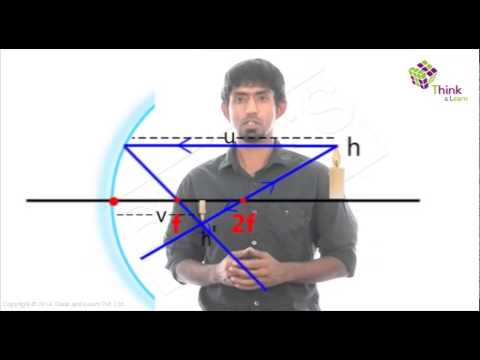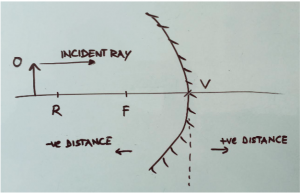Checkout JEE MAINS 2022 Question Paper Analysis : Checkout JEE MAINS 2022 Question Paper Analysis :

# Mirror Formula For Spherical Mirrors

An object placed in front of a mirror generates an image. If light rays from the object fall on the mirror and are then reflected and converge to form an image, the image thus formed is a real image. If the reflected light rays do not converge but have to be extrapolated backwards to form an image, the image is called a virtual image.

In this article, we shall discuss the mirror formula, which is often taught and frequently used in various instances. As such, using ray diagrams, it is possible to determine the type of image formed, while using concave and convex mirrors, based on the distance of the object from the mirror. To obtain exact information about the size and magnification of the image, and the distance of the image from the spherical mirror, we can use the mirror formula.

## What is Mirror Formula?

The Mirror Formula (also referred to as the mirror equation) gives us the relationship between the focal length (f), the distance of the object from the mirror (u) and the distance of the image from the mirror (v). The mirror formula for a concave mirror is given below. The magnification image formed by a spherical mirror is given by the height of the image divided by the height of the object. The formula is given as:

$$\begin{array}{l}\frac{1}{f}=\frac{1}{v}+\frac{1}{u}\end{array}$$## Sign Convention for Spherical Mirrors

• Distances are to be measured from the pole (vertex) of the mirror marked by point V in the figure.
• Distances measured along the direction of the incident ray are positive. The distance measured opposite the direction of the incident ray is negative.
• Distances measured above the principal axis are positive. Distances measured below the principal axis are negative.

The above convention applies to both concave and convex mirrors and spherical mirrors. The figure below shows a concave mirror, but the same applies to a convex mirror as well.Following is the table explaining concepts related to mirror:

## Solved Examples

Here are some examples of spherical mirror formula problems:

1) A lens having a focal distance of 30 cm is placed in front of an object, which is located at 1 m from it. Where is an image of the object located?

From the image position formula:

1/f = 1/o + 1/I or 1/o + 1/I = 1/f

1/(100 cm) + 1/I = 1/(30 cm)

1/I =0.023/cm

I = 42.85 cm

2) A lens forms an image at 25 cm from it, where the real object is placed at 1 m. What is the focal length of the lens and its curvature radius?

From the image position formula, we find:

1/f = 1/o + 1/I = (o+I)/(o*I)

f = o*I/(o+I) = 25 cm * 100 cm2 /(125 cm)

f = 2500/125 cm = 20 cm

then the curvature radius is r ≈ 2 * f = 40 cm

## Frequently Asked Questions – FAQs

### How do convex mirrors work?

A convex mirror is a diverging mirror in which the reflective surface bulges towards the light source. They are not used to focus light as they reflect light outwards. The image formed by convex mirrors is smaller than the object but gets larger as they approach the mirror.

### How do concave mirrors work?

A concave mirror has a reflective surface that is curved inward and away from the light source. Concave mirrors reflect light inward to one focal point. Unlike convex mirrors, the image formed by a concave mirror shows different image types depending on the distance between the object and the mirror.

### What is Mirror Formula?

The Mirror Formula (also referred to as the mirror equation) gives us the relationship between the focal length (f), the distance of the object from the mirror (u) and the distance of the image from the mirror (v).
$$\begin{array}{l}\frac{1}{f} = \frac{1}{v} + \frac{1}{u}\end{array}$$

### For a concave mirror, the image formed has a negative magnification sign. What is the feature of the image formed?

We know that for a concave mirror, magnification is the ratio of the height of the image formed to the height of the object. Since the magnification is negative, that means the height of the image formed is below the principal axis and hence the image formed is Real and Inverted.

### What is the value of magnification when the image size is double that of the object size?

Magnification is the ratio of the height of the image formed to the height of the object. Thus, when the image size is double that of the object then the magnification would be 2.

Stay tuned with BYJU’S for more such interesting articles. Also, register to “BYJU’S – The Learning App” for loads of interactive, engaging Physics-related videos and an unlimited academic assist.